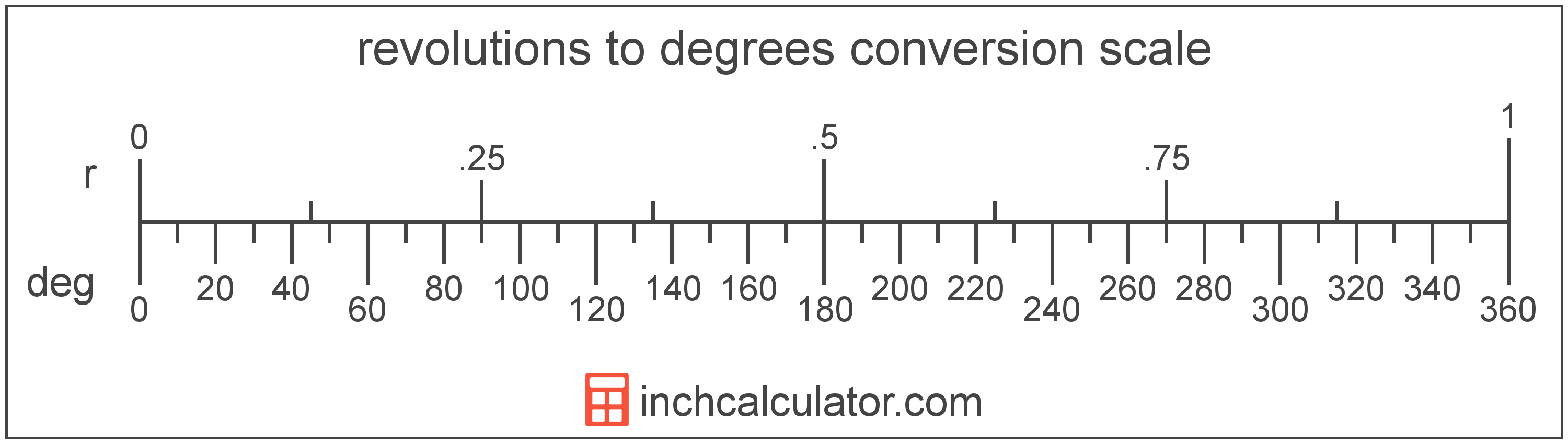# Revolutions to Degrees Converter

Enter the angle in revolutions below to get the value converted to degrees.

Results in Degrees:1 r = 360°
1 r = 360° 0′ 0″

Do you want to convert degrees to revolutions?

## How to Convert Revolutions to Degrees

To convert a measurement in revolutions to a measurement in degrees, multiply the angle by the following conversion ratio: 360 degrees/revolution.

Since one revolution is equal to 360 degrees, you can use this simple formula to convert:

degrees = revolutions × 360

The angle in degrees is equal to the angle in revolutions multiplied by 360.

For example, here's how to convert 5 revolutions to degrees using the formula above.
degrees = (5 r × 360) = 1,800°### How Many Degrees Are in a Revolution?

There are 360 degrees in a revolution, which is why we use this value in the formula above.

1 r = 360°

## What Is a Revolution?

A revolution, or turn, is equal to 1 rotation around a circle, or 360°. Revolutions are commonly used to measure the speed of rotation, for example when measuring the revolutions per minute (RPM) of a vehicle's engine.

A revolution is sometimes also referred to as a turn, cycle, or complete rotation. Revolutions can be abbreviated as r, and are also sometimes abbreviated as rev or cyc. For example, 1 revolution can be written as 1 r, 1 rev, or 1 cyc.

## What Is a Degree?

A degree is a measure of angle equal to 1/360th of a revolution, or circle. The number 360 has 24 divisors, making it a fairly easy number to work with. There are also 360 days in the Persian calendar year, and many theorize that early astronomers used 1 degree per day.

The degree is an SI accepted unit for angle for use with the metric system. A degree is sometimes also referred to as a degree of arc, arc degree, or arcdegree. Degrees can be abbreviated as °, and are also sometimes abbreviated as deg. For example, 1 degree can be written as 1° or 1 deg.

Degrees can also be expressed using arcminutes and arcseconds as an alternative to using the decimal form. Arcminutes and arcseconds are expressed using the prime (′) and double-prime (″) characters, respectively, although a single-quote and double-quote are often used for convenience.

One arcminute is equal to 1/60th of a degree, and one arcsecond is equal to 1/60th of an arcminute.

Protractors are commonly used to measure angles in degrees. They are semi-circle or full-circle devices with degree markings allowing a user to measure an angle in degrees. Learn more about how to use a protractor or download a printable protractor.

## Revolution to Degree Conversion Table

Table showing various revolution measurements converted to degrees.
Revolutions Degrees
1 r 360°
2 r 720°
3 r 1,080°
4 r 1,440°
5 r 1,800°
6 r 2,160°
7 r 2,520°
8 r 2,880°
9 r 3,240°
10 r 3,600°
11 r 3,960°
12 r 4,320°
13 r 4,680°
14 r 5,040°
15 r 5,400°
16 r 5,760°
17 r 6,120°
18 r 6,480°
19 r 6,840°
20 r 7,200°
21 r 7,560°
22 r 7,920°
23 r 8,280°
24 r 8,640°
25 r 9,000°
26 r 9,360°
27 r 9,720°
28 r 10,080°
29 r 10,440°
30 r 10,800°
31 r 11,160°
32 r 11,520°
33 r 11,880°
34 r 12,240°
35 r 12,600°
36 r 12,960°
37 r 13,320°
38 r 13,680°
39 r 14,040°
40 r 14,400°

## References

1. Collins Dictionary, Definition of 'degree', https://www.collinsdictionary.com/us/dictionary/english/degree